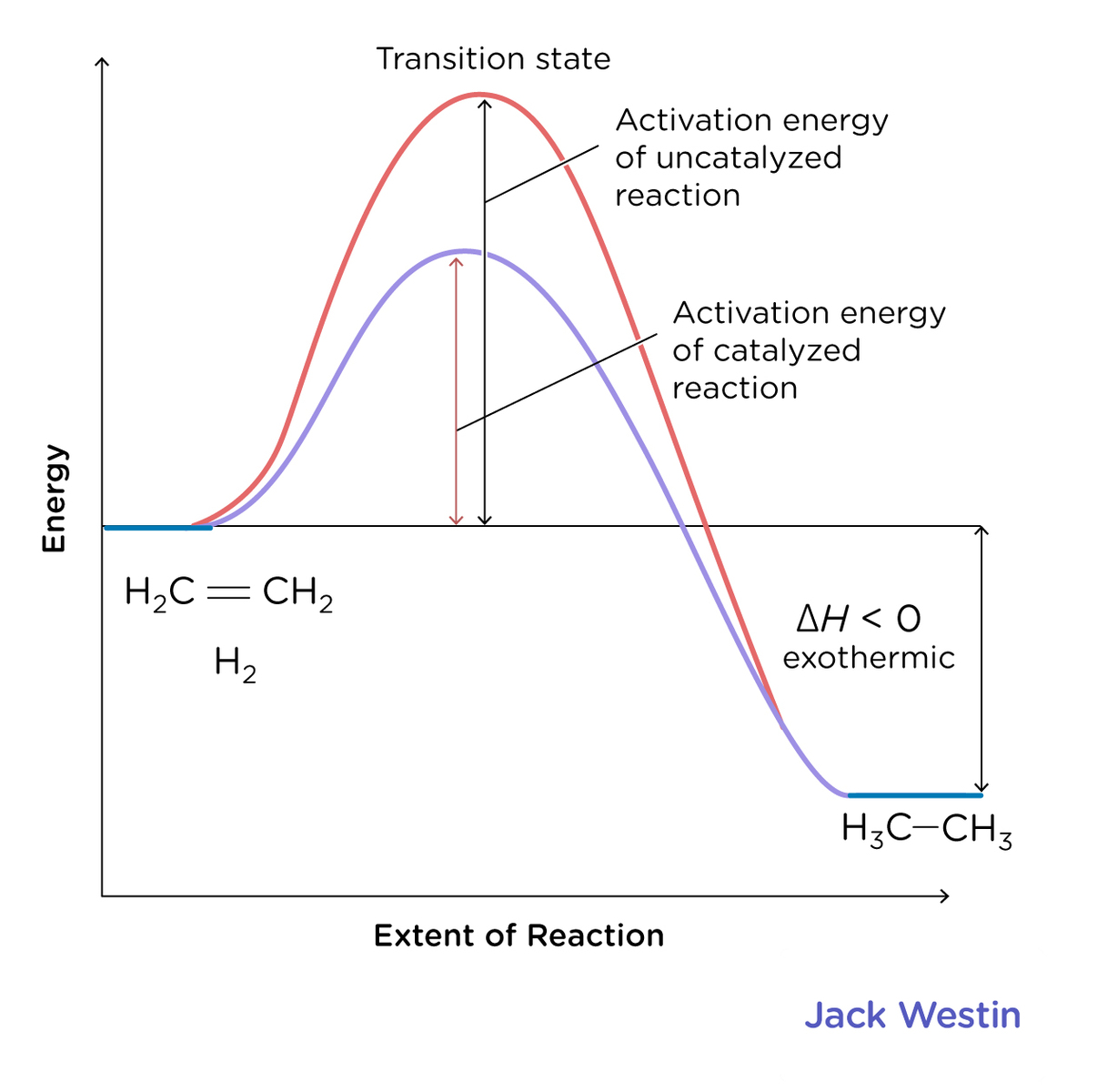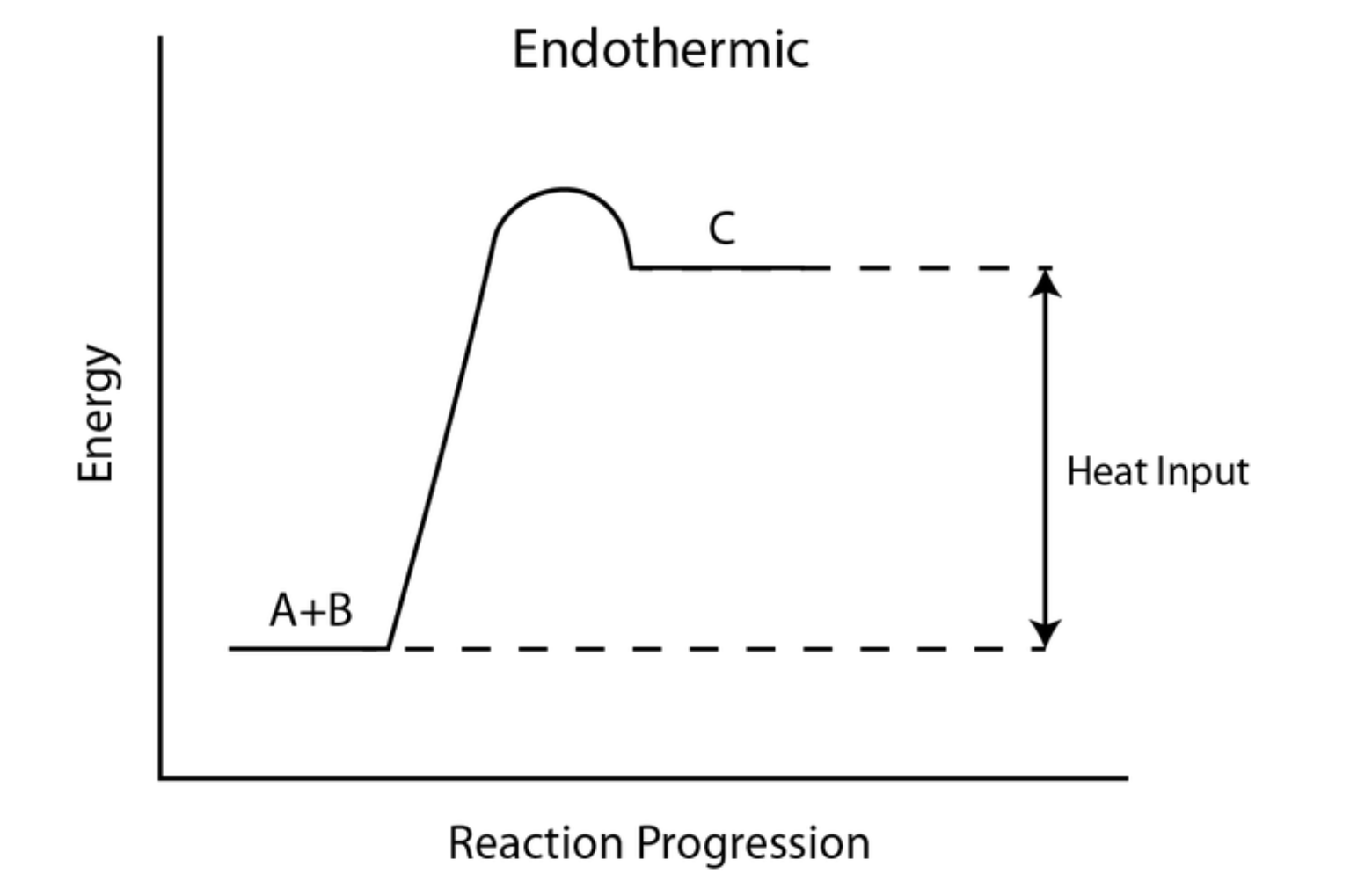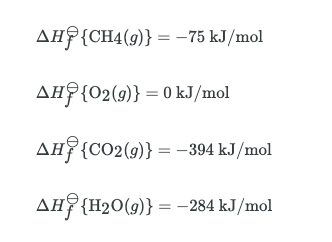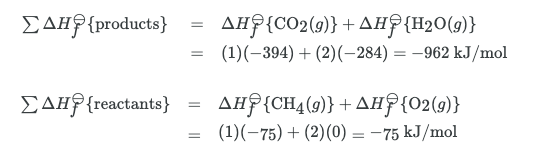Complimentary 1-hour tutoring consultation

MCAT Content / Energy Changes In Chemical Reactions / Endothermic Exothermic Reactions

### Endothermic/Exothermic Reactions

Topic: Energy Changes In Chemical Reactions

Reactions can either release or absorb energy in the form of heat; Endothermic reactions absorb heat while exothermic reactions release heat.

The absorption and release of heat can be represented quantitatively by enthalpy (H), which equates to the heat content of a system. A positive +ΔH value results in a gain of heat in a system, which represents an endothermic system due to the absorbance of heat. A negative -ΔH value results in the loss of heat in a system, which represents an exothermic system due to the release of heat. This can be represented by energy level diagrams which show the relative enthalpies of reactants and products and the change in enthalpy as a result.Enthalpy can be represented by Joules over moles, or J/mol.

Enthalpy changes can be calculated by calculating the standard enthalpy of reaction, ΔHrxn. This is the change in heat content for any reaction and this can be calculated by calculating the sum enthalpy of the products and subtracting it from the sum enthalpy of the reactants.

Enthalpy changes can also be calculated using the standard enthalpy of formation, ΔHf, is the change in enthalpy that accompanies the formation of one mole of the compound from its elements in their standard states. For example, the standard enthalpy of formation for carbon dioxide would be the change in enthalpy for the following reaction:Hess’s law can be used to calculate the enthalpy of reaction using the sum of the standard enthalpies of the intermediate reactions into which the overall reaction can be divided, while each occurs at the same temperature. Hess’s law is based on the fact that the enthalpy change for a reaction is independent of the number of ways a product can be obtained if the initial and final conditions are the same.Graphical representation of Hess’s law: The net reaction here is A being converted into D, and the change in enthalpy for that reaction is ΔH. However, we can see that the net reaction is a result of A being converted into B, which is then converted into C, which is finally converted into D. By Hess’s law, the net change in enthalpy of the overall reaction is equal to the sum of the changes in enthalpy for each intermediate transformation: ΔH = ΔH1+ΔH2+ΔH3.

A negative enthalpy change for a reaction indicates exothermic process, while a positive enthalpy change corresponds to endothermic process.In order to calculate the standard enthalpy of reaction, we need to look up the standard enthalpies of formation for each of the reactants and products involved in the reaction. These are typically found in an appendix or in various tables online. For this reaction, the data we need is:Note that because it exists in its standard state, the standard enthalpy of formation for oxygen gas is 0 kJ/mol. Next, we sum up our standard enthalpies of formation. Keep in mind that because the units are in kJ/mol, we need to multiply by the stoichiometric coefficients in the balanced reaction equation.Now, we can find the standard enthalpy of reaction:Practice Questions

MCAT Official Prep (AAMC)

Chemistry Question Pack Passage 11 Question 62

Key Points

• Enthalpy (ΔH) represents the heat content in a system.

• Exothermic reactions release heat (-ΔH) and endothermic reactions absorb heat (+ΔH)

• Hess’s law states that the standard reaction enthalpy is the sum of the standard enthalpies of the intermediate reactions into which the overall reaction can be divided, while each occurs at the same temperature.

• The standard enthalpy of reaction, , can be calculated by summing the standard enthalpies of formation of the reactants and subtracting the value from the sum of the standard enthalpies of formation of the products.

Key Terms

exothermic: net release of heat.

endothermic: net absorbance of heat

enthalpy: in thermodynamics, a measure of the heat content of a chemical or physical system

standard enthalpy of formation: the change in enthalpy that accompanies the formation of one mole of a compound from its elements, with all substances in their standard states

standard enthalpy of reaction: the enthalpy change that occurs in a system when one mole of matter is transformed by a chemical reaction under standard conditions

Hess’s law: states that, if an overall reaction takes place in several steps, its standard reaction enthalpy is the sum of the standard enthalpies of the intermediate reactions, at the same temperature.

Billing Information